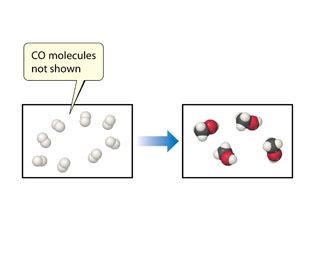# Problem: The following diagram shows the combination reaction between hydrogen, H2, and carbon monoxide, CO, to produce methanol, CH3OH (white spheres are H, black spheres are C, red spheres are O). The correct number of CO molecules involved in this reaction is not shown.Determine the number of CO molecules that should be shown in the left (reactants) box.

###### FREE Expert Solution

In this problem, we are asked to determine the number of CO molecules that should be shown in the left (reactants) box.

Before balancing the chemical reaction, let’s first write the chemical formula of each of the compounds involved in the reaction.

Reactants:

H2

▪ CO

99% (38 ratings)###### Problem Details

The following diagram shows the combination reaction between hydrogen, H2, and carbon monoxide, CO, to produce methanol, CH3OH (white spheres are H, black spheres are C, red spheres are O). The correct number of CO molecules involved in this reaction is not shown.Determine the number of CO molecules that should be shown in the left (reactants) box.